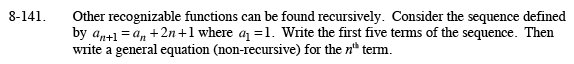### Home > PC > Chapter 8 > Lesson 8.2.5 > Problem8-141

8-141.

Other recognizable functions can be found recursively. Consider the sequence defined by an+1 = an + 2n + 1 where a1 = 1. Write the first five terms of the sequence. Then write a general (non-recursive) equation for the nth term.. Homework Help ✎an+1= an + 2n + 1
a1 = 1
a2 = 1+ 2(1) + 1 = 4
a3 = 4 + 2(2) + 1 = 9
a4 = 9 + 2(3) + 1 = 16
a5 = 16 + 2(4) + 1 = 25

an = n2